Latest Banking jobs   »   Quantitative Aptitude Quiz For SBI Clerk...

# Quantitative Aptitude Quiz For SBI Clerk Prelims 2021- 17th May

Directions (1-5): In the following questions two quantities are given for each question. Compare the numeric value of both the quantities and answers accordingly
Q1. Ravi sent ‘X’ messages in a day, 20% in which sent to Ram. Out of remaining 25% sent to Shyam. Remaining messages sent to Rahul and Gopal is in the ratio 4 : 5. Rahul got 24 message.
Quantity I: Value of ‘1.5X’
Quantity II: ‘X + 35’
(a) Quantity I > Quantity II
(b) Quantity I < Quantity II
(c) Quantity I ≥ Quantity II
(d) Quantity I ≤ Quantity II
(e) Quantity I = Quantity II or No relation

Q2. Area of a square of side 16 cm is same as area of a rectangle whose breadth is 8 cm.
Quantity I: Perimeter of square.
Quantity II: Perimeter of rectangle.
(a) Quantity I > Quantity II
(b) Quantity I < Quantity II
(c) Quantity I ≥ Quantity II
(d) Quantity I ≤ Quantity II
(e) Quantity I = Quantity II or No relation

Q3. Train ‘A’ can cross a pole in 20 seconds and a platform which is 60 m long in 25 seconds. Train ‘A’ can cross train ‘B’ coming from opposite direction in 15 seconds. Ratio between speed of train ‘A’ to train ‘B’ is 2 : 3.
Quantity I: Length of train ‘B’
Quantity II: Length of train ‘A’
(a) Quantity I > Quantity II
(b) Quantity I < Quantity II
(c) Quantity I ≥ Quantity II
(d) Quantity I ≤ Quantity II
(e) Quantity I = Quantity II or No relation

Q4. Probability of selecting one yellow ball out of a box consisting some yellow balls and some green balls is 4/7.
Quantity I: No. of yellow balls in box.
Quantity II: No. of green balls in box.
(a) Quantity I > Quantity II
(b) Quantity I < Quantity II
(c) Quantity I ≥ Quantity II
(d) Quantity I ≤ Quantity II
(e) Quantity I = Quantity II or No relation

Q5. Quantity I : Distance covered in upstream in 10.8 hours
Ratio of upstream speed to downstream speed is 1 : 11. If speed of boat in still water is 30 km/hr.
Quantity II : Find x.
The time taken by a boat for covering ‘x – 18’ km upstream is equal to time taken by it for covering ‘x’ km downstream. Upstream speed is 6 km/hr less than downstream speed and speed of boat in still water in 15 kmph.
(a) Quantity I > Quantity II
(b) Quantity II > Quantity I
(c) Quantity I ≥ Quantity II
(d) Quantity II ≥ Quantity I
(e) Quantity I = Quantity II or relation can’t be established

Directions (6-10): In the following questions two quantities are given for each question. Compare the numeric value of both the quantities and answers accordingly
Q6. Ram invested Rs80000 for a year and Shyam invested Rs72000 For 8 months.
Quantity I : If total profit at the end of year is Rs11400 then the share of Ram.
Quantity II : Rs. 1420
(a) Quantity I > Quantity II
(b) Quantity II > Quantity I
(c) Quantity I ≥ Quantity II
(d) Quantity II ≥ Quantity I
(e) Quantity I = Quantity II or relation can’t be established

Q7. 24 men can do a work in 20 days and 36 women can do the same work in 24 days
Quantity I : Time taken by 12 man to complete whole work
Quantity II : Time taken by 16 women to complete whole work.
(a) Quantity I > Quantity II
(b) Quantity II > Quantity I
(c) Quantity I ≥ Quantity II
(d) Quantity II ≥ Quantity I
(e) Quantity I = Quantity II or relation can’t be established

Q8. Average age of A, B and C is 37 years and average age of A and B is 23 years
Quantity I : What is the age of C
Quantity II : 65 years
(a) Quantity I > Quantity II
(b) Quantity II > Quantity I
(c) Quantity I ≥ Quantity II
(d) Quantity II ≥ Quantity I
(e) Quantity I = Quantity II or relation can’t be established

Q9. A train of length 360 m is travelling at a speed of 54 km/hr
Quantity I : Find the time take by train to cross a pole
Quantity II : If its speed is increased by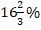then find the time in which it can cross a platform of length 130 m
(a) Quantity I > Quantity II
(b) Quantity II > Quantity I
(c) Quantity I ≥ Quantity II
(d) Quantity II ≥ Quantity I
(e) Quantity I = Quantity II or relation can’t be established

Q10. Quantity I : A sphere of diameter 13.4 cm is melted and cast into a right circular cone of height 26.8 cm. The radius of the base of the cone is ?
Quantity II: 5.95 cm.
(a) Quantity I > Quantity II
(b) Quantity II > Quantity I
(c) Quantity I ≥ Quantity II
(d) Quantity II ≥ Quantity I
(e) Quantity I = Quantity II or relation can’t be established

Directions (11-13): The following questions are accompanied by two statements (I) and (II). You have to determine which statements(s) is/are sufficient/necessary to answer the questions.
(a) Statement I alone is sufficient to answer the question but statement II alone is not sufficient to answer the questions.
(b) Statement II alone is sufficient to answer the question but statement I alone is not sufficient to answer the question.
(c) Both the statements taken together are necessary to answer the questions, but neither of the statements alone is sufficient to answer the question.
(d) Either statement I or statement II by itself is sufficient to answer the question.
(e) Statements I and II taken together are not sufficient to answer the question.

Q11. Find the age of Chauhan if minimum age difference between the age of any two persons (out of Abhi, Billi & Chauhan) is 2 years.
(i) Ratio of age of Chauhan to Billi is 3 : 2.
(ii) Ratio of age of Abhi 6 years ago to age of Billi 2 years hence is 1 : 2.

Q12. Calculate the rate of interest
(i) An amount of Rs. 864 is obtained at the principal of Rs. 800 at SI.
(ii) An amount of Rs. 176 is obtained after 19 years when Rs. 100 is submitted at SI.

Q13. What is the area of equilateral ∆ABC.
(i) The height of triangle is 3√3 cm.
(ii) Ratio of area of triangle ABC to area of similar triangle PQR is 9 : 4.

Directions (14-15): The following questions are accompanied by two statements A and B. You have to determine which statements(s) is/are sufficient/necessary to answer the questions.
(a) Both the statements taken together are necessary to answer the questions, but neither of the statements alone is sufficient to answer the question.
(b) Statement B alone is sufficient to answer the question, but statement A alone is not sufficient to answer the question.
(c) Either statement A or statement B by itself is sufficient to answer the question.
(d) Statement A alone is sufficient to answer the question, but statement B alone is not sufficient to answer the questions.
(e) Statements A and B taken together are not sufficient to answer the question.

Q14. Find Veer’s present age if Atul is ten year younger than Veer.
(A) Five year hence, Atul’s age is 20% more than Abhi’s age while Abhi is 15 years younger than Veer.
(B) Ratio between Veer’s present age to Atul present age is 7 : 5

Q15. Find the speed of boat in downstream?
(A) Speed of boat in still water is 50% more than speed of boat in upstream.
(B) Difference between time taken by boat to cover 32 km in upstream to that of in downstream is 2 hours.

Practice More Questions of Quantitative Aptitude for Competitive Exams:

###### SBI Clerk Study Plan 2021

Solutions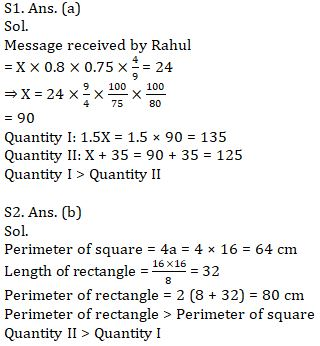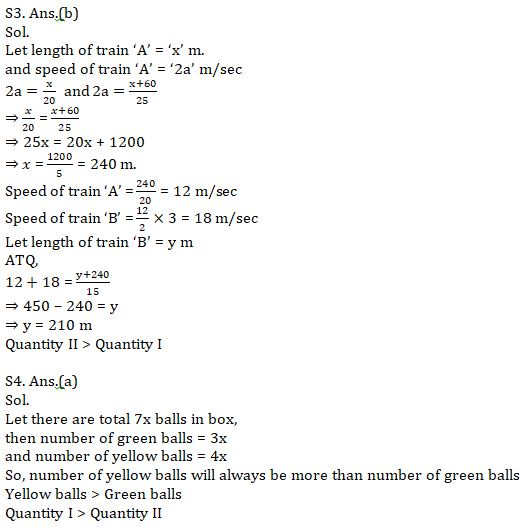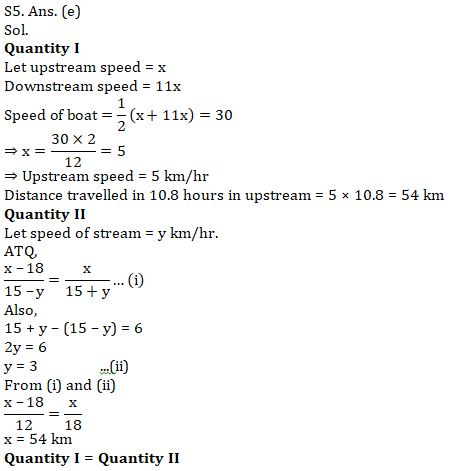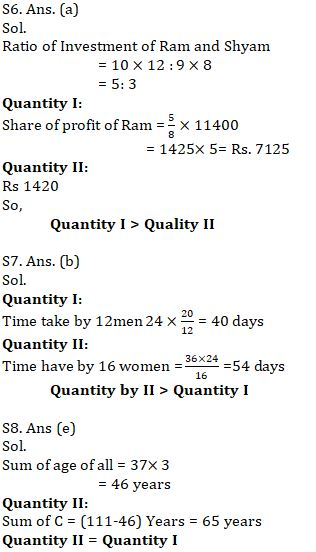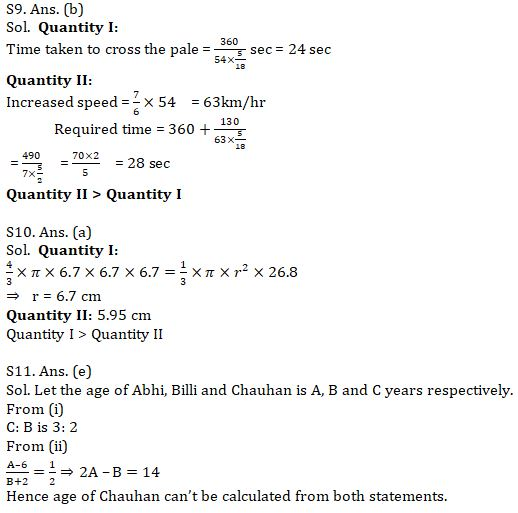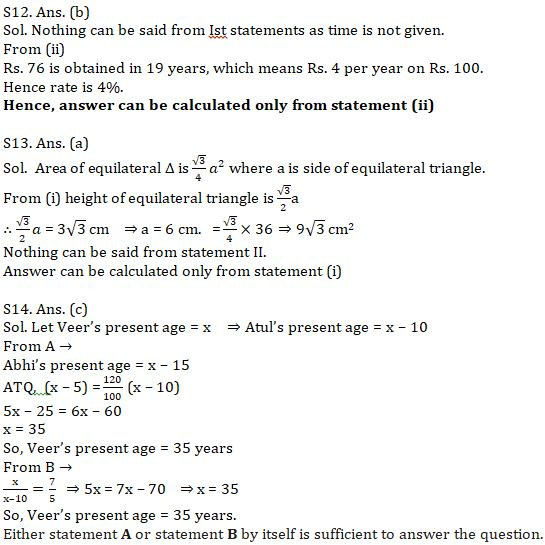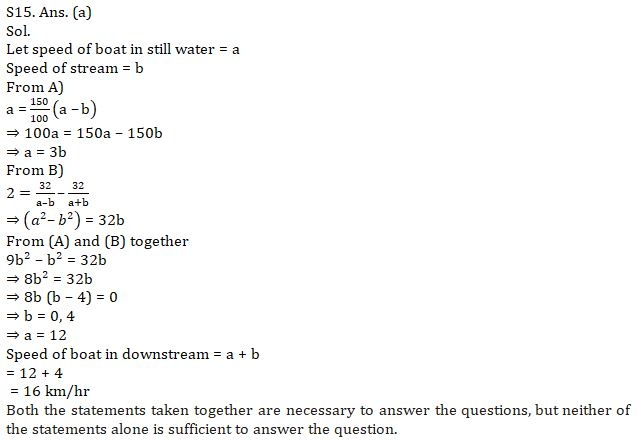#### Congratulations!